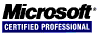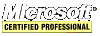## The C++ Smart Pointer

A smart/managed pointer is an object that provides automatic memory management features over raw/traditional pointers. Smart pointer objects are placed on the runtime stack and when they go out of scope they are destroyed along with the memory of the managed object if there are no other references to the object. The managed object's destructor is called, too.

Shared pointers (shared_ptr) have no limit on the number of aliases that can reference an object. When the reference count reaches zero the object's destructor is called. Shared pointers occupy 8 bytes on the runtime stack of a 32-bit system. When working with nodes, shared pointers are better than unique or weak pointers. What is happening behind the scenes is that the pointers point to the same Manager object which has the reference count stored. The Manager object then has a pointer to the managed object.

Weak pointers (weak_ptr) store a reference to an object managed by shared pointers. These pointers do not affect the reference count for the object. The size of this pointer is eight bytes on a 32-bit system.

Unique pointers (unique_ptr) have no copy constructor and have to be passed by reference. They can not be assigned to another pointer, but they can be moved using the `std::move()` function. Unique pointers occupy only four bytes on the runtime stack of a 32-bit system.

On 64-bit systems, pointer sizes double.

``````#include <memory>
#include <iostream>
#include <string>
using namespace std;

template <class TData> class clsOne
{
private:
TData data;
public:
clsOne() {}
clsOne(TData &param) : data(param) {}
~clsOne() {}
const TData &getData() const { return data; }
};

class clsTwo
{
private:
string data;
public:
clsTwo() {}
clsTwo(string &param) : data(param) {}
~clsTwo() {}
const string &getData() const { return data; }
};

void func_shared(shared_ptr<clsTwo> ptr)
{
cout << "reference count: " << ptr.use_count() << endl;
cout << ptr->getData() << endl;
}

void func_unique(unique_ptr<int[]> &ptr)
{
cout << ptr << endl;
}

int main()
{
string str = "testA";
shared_ptr<clsOne<string>> ptr1 = make_shared<clsOne<string>>(str);
shared_ptr<clsOne<string>> ptr2 = ptr1; // use the copy constructor
cout << ptr2->getData() << endl;

str = "testB";
shared_ptr<clsTwo> ptr3 = make_shared<clsTwo>(str);
cout << "reference count: " << ptr3.use_count() << endl;
cout << ptr3->getData() << endl;

func_shared(ptr3);
cout << "reference count: " << ptr3.use_count() << endl;

cout << "sizeof shared_ptr: " << sizeof(ptr3) << endl;

int n = 90210;
shared_ptr<clsOne<int>> ptr4(new clsOne<int>(n));
cout << "reference count: " << ptr3.use_count() << endl;
cout << ptr4->getData() << endl;

cout << "sizeof shared_ptr: " << sizeof(ptr4) << endl;

unique_ptr<int[]> ptr5(new int);
for (int i = 0; i < 5; ptr5[i] = i++);
for (int i = 0; i < 5; i++)
cout << ptr5[i];

cout << endl << "sizeof unique_ptr: " << sizeof(ptr5) << endl;

ptr5 = (unique_ptr<int[]>)new int;
for (int i = 0; i < 10; ptr5[i] = i++);
for (int i = 0; i < 10; i++)
cout << ptr5[i];

cout << endl << "sizeof unique_ptr: " << sizeof(ptr5) << endl;

func_unique(ptr5);

ptr5.release(); // manually delete

cout << "reference count: " << ptr3.use_count() << endl;
weak_ptr<clsTwo> ptr6 = ptr3;
cout << "reference count: " << ptr3.use_count() << endl;

cout << endl << "sizeof weak_ptr: " << sizeof(ptr6) << endl;

return 0;
}
``````